# A Simple Model

## Simple Model for Packet Forwarding

Network

• network is a path of $n$ buffers
• packets have common destination: rightmost node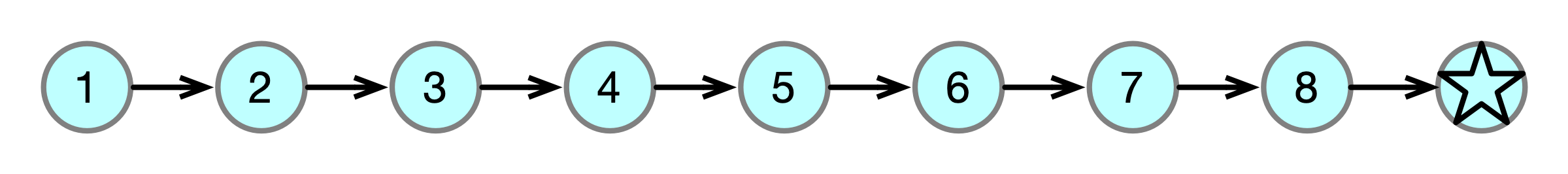Execution in synchronous rounds

• 1 packet injected per round (arbitrary buffer)
• each buffer can forward at most one packet per round

Goal. Deliver packets using minimum space per buffer

## Previous Work

1. For centralized protocols, buffer space 2 is necessary and sufficient
• Forward-if-empty protocol
• Miller & Patt-Shamir, DISC 2016
2. For local protocols, buffer space $\Theta(\log n)$ is necessary and sufficient
• Odd-Even Downhill (OED) protocol
• Dobrev et al., SPAA 2017; Patt-Shamir & R– PODC 2017
3. For protocols with locality $d$, buffer space $\Theta(1 + \frac 1 d \log n)$ is necessary and sufficient
• Patt-Shamir & R– INFOCOM 2019

## Generalizing the Model: AQT

Adversarial Queueing Theory (Borodin et al. STOC 1996)

• rate $\rho$
• burst parameter $\sigma$
• edge capacity $C$

Executions

• in any $t$ consecutive rounds, adversary can inject $\rho t + \sigma$ packets
• each buffer can forward $C (\geq \rho)$ packets

## Previous Results in AQT Model

1. For cenralized protocols, buffer space $2 \rho + \sigma$ is necessary and sufficient

2. For local protocols, buffer space $\Theta(\rho \log n + \sigma)$ is necessary and sufficient (?)

3. For protocols with locality $d$, buffer space $\Theta(1 + \frac \rho d \log n + \sigma)$ is necessary and sufficient

• ugly algorithm/analysis for $C > 1$

# Local Bursts: Refining the AQT Model

## Global Bursts

AQT parameters do not distinguish ($\rho = 1, \sigma = n - 1$):

• Ex 1 every $n$ rounds, inject $n$ packets into buffer $1$

• Ex 2 every $n$ rounds, injection $1$ packet into buffers $1, 2, \ldots, n$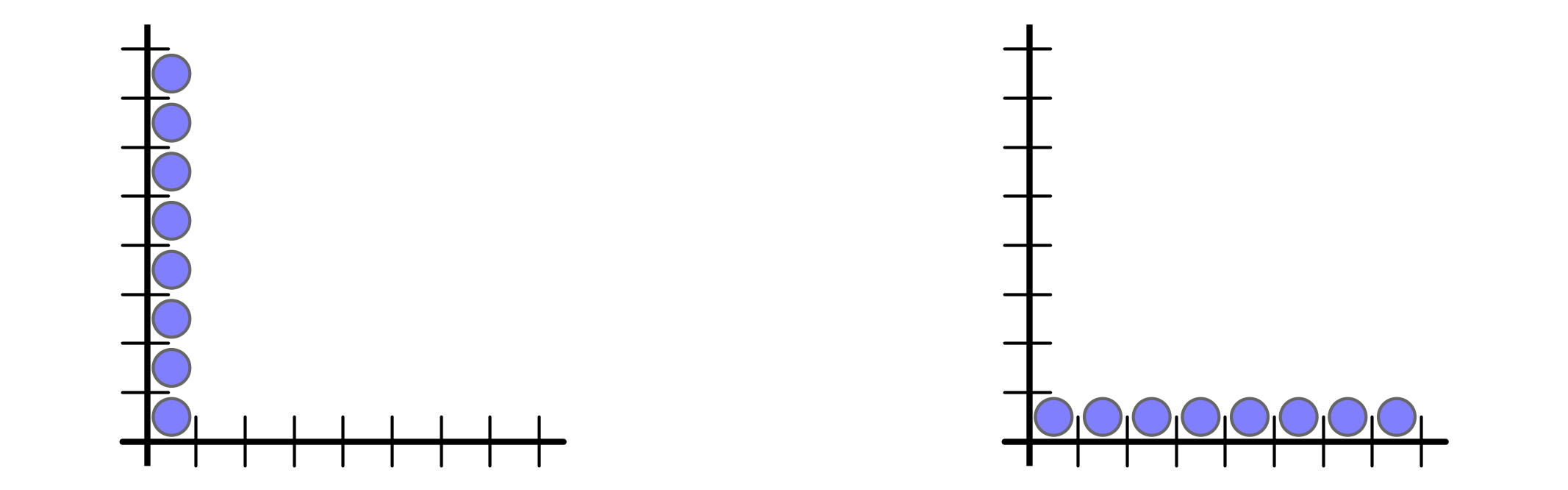But buffer space requirement is $n$ vs $1$

## Global Bursts

AQT parameters do not distinguish ($\rho = 1, \sigma = n - 1$):

• Ex 1 every $n$ rounds, inject $n$ packets into buffer $1$

• Ex 2 every $n$ rounds, injection $1$ packet into buffers $1, 2, \ldots, n$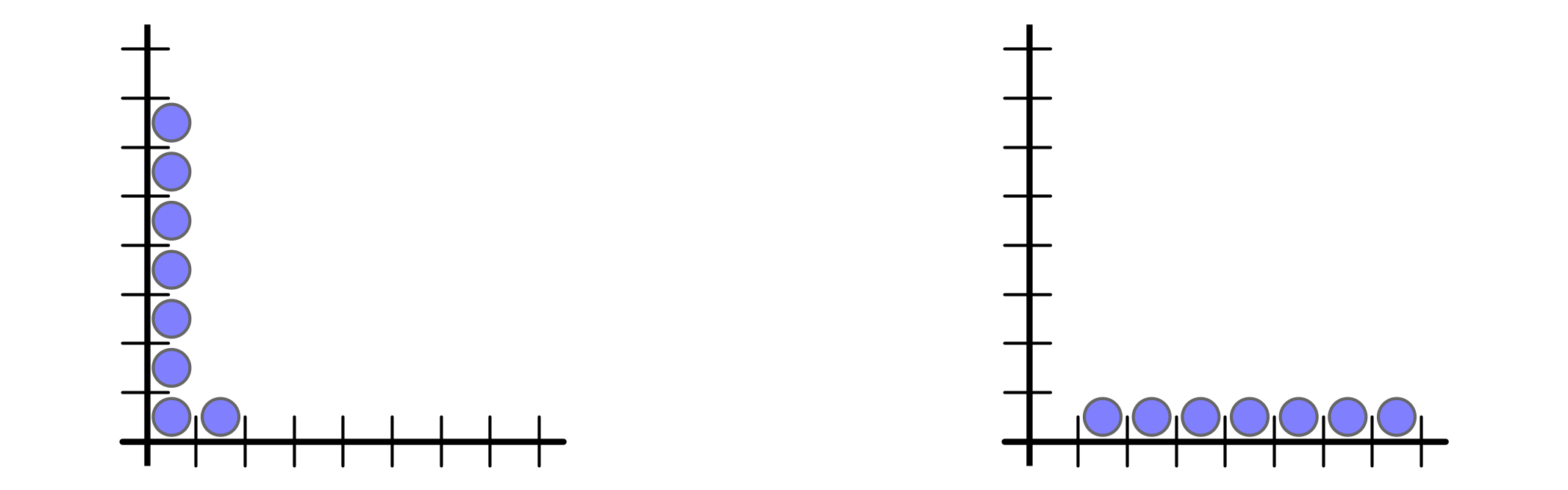But buffer space requirement is $n$ vs $1$

## Global Bursts

AQT parameters do not distinguish ($\rho = 1, \sigma = n - 1$):

• Ex 1 every $n$ rounds, inject $n$ packets into buffer $1$

• Ex 2 every $n$ rounds, injection $1$ packet into buffers $1, 2, \ldots, n$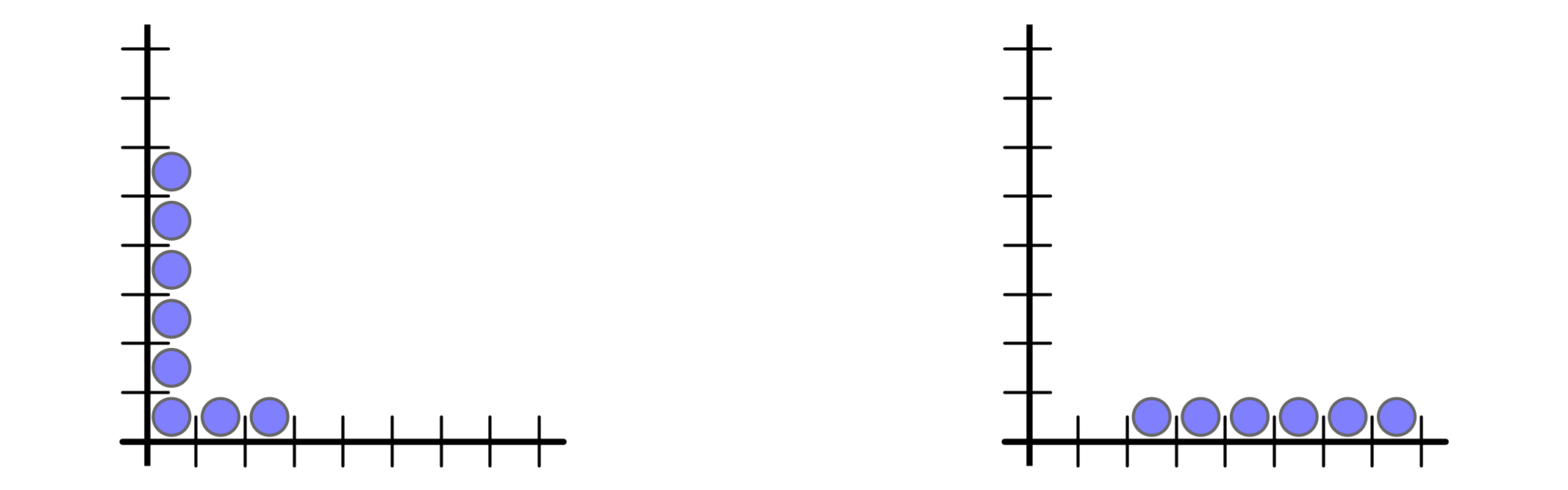But buffer space requirement is $n$ vs $1$

## Global Bursts

AQT parameters do not distinguish ($\rho = 1, \sigma = n - 1$):

• Ex 1 every $n$ rounds, inject $n$ packets into buffer $1$

• Ex 2 every $n$ rounds, injection $1$ packet into buffers $1, 2, \ldots, n$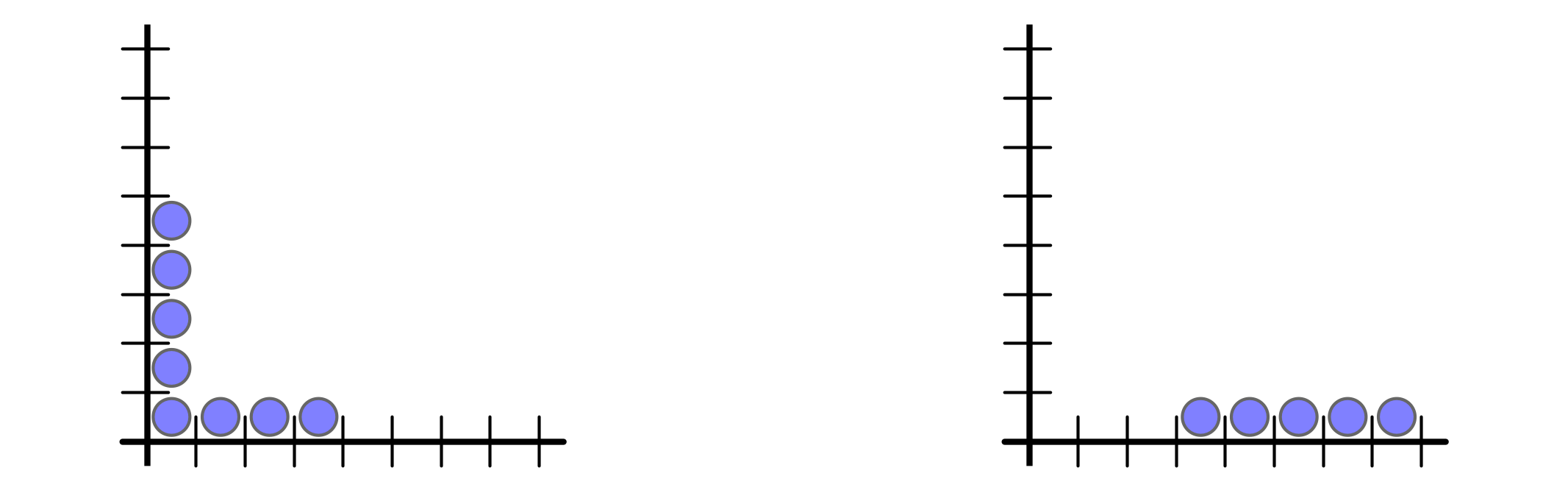But buffer space requirement is $n$ vs $1$

## Global Bursts

AQT parameters do not distinguish ($\rho = 1, \sigma = n - 1$):

• Ex 1 every $n$ rounds, inject $n$ packets into buffer $1$

• Ex 2 every $n$ rounds, injection $1$ packet into buffers $1, 2, \ldots, n$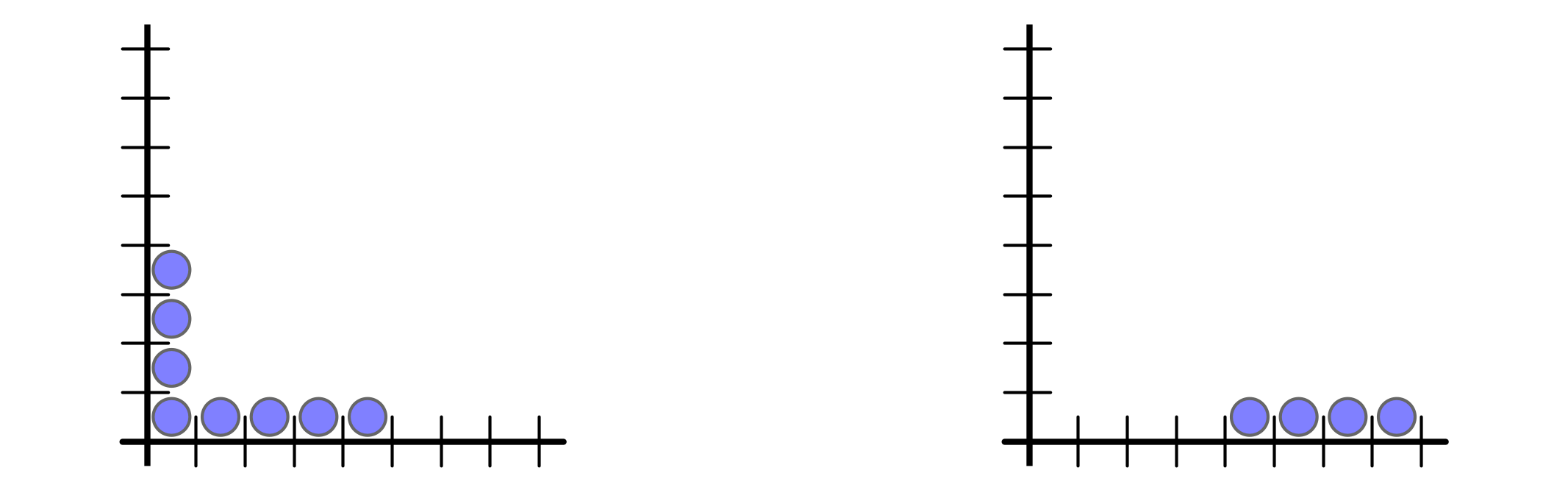But buffer space requirement is $n$ vs $1$

## Global Bursts

AQT parameters do not distinguish ($\rho = 1, \sigma = n - 1$):

• Ex 1 every $n$ rounds, inject $n$ packets into buffer $1$

• Ex 2 every $n$ rounds, injection $1$ packet into buffers $1, 2, \ldots, n$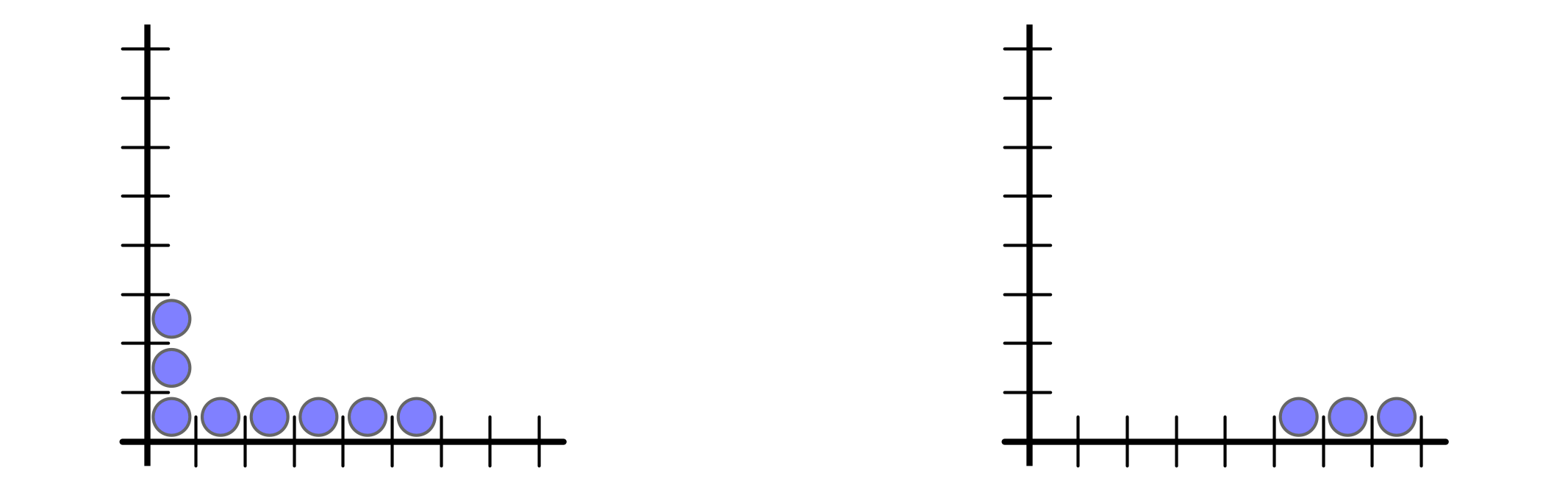But buffer space requirement is $n$ vs $1$

## Global Bursts

AQT parameters do not distinguish ($\rho = 1, \sigma = n - 1$):

• Ex 1 every $n$ rounds, inject $n$ packets into buffer $1$

• Ex 2 every $n$ rounds, injection $1$ packet into buffers $1, 2, \ldots, n$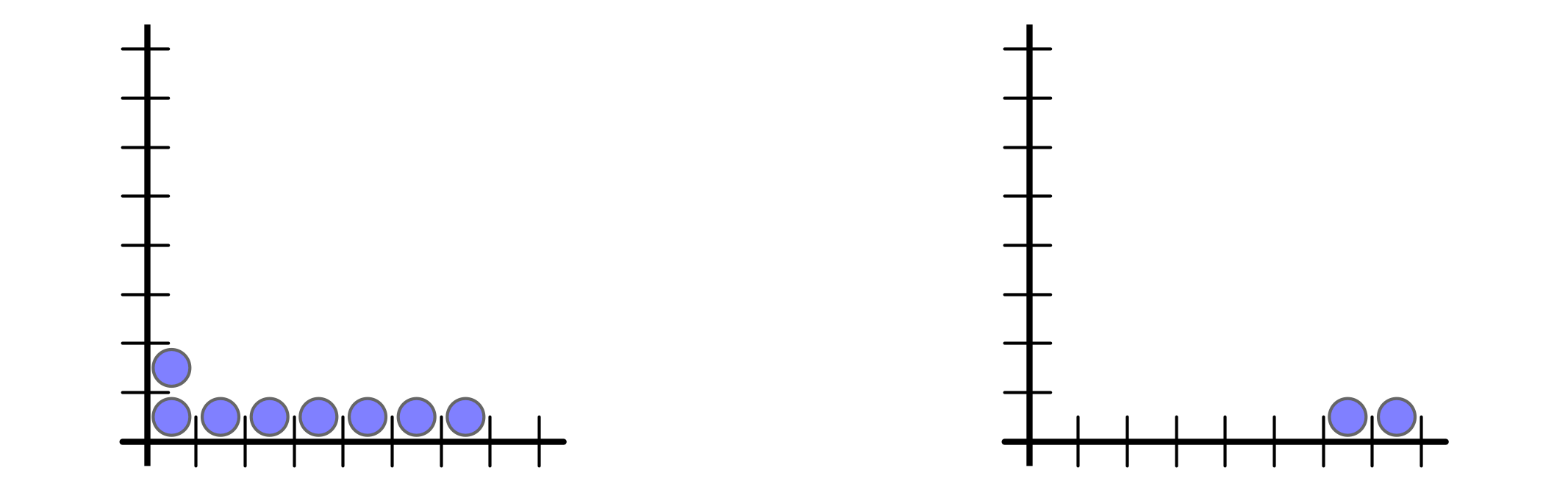But buffer space requirement is $n$ vs $1$

## Global Bursts

AQT parameters do not distinguish ($\rho = 1, \sigma = n - 1$):

• Ex 1 every $n$ rounds, inject $n$ packets into buffer $1$

• Ex 2 every $n$ rounds, injection $1$ packet into buffers $1, 2, \ldots, n$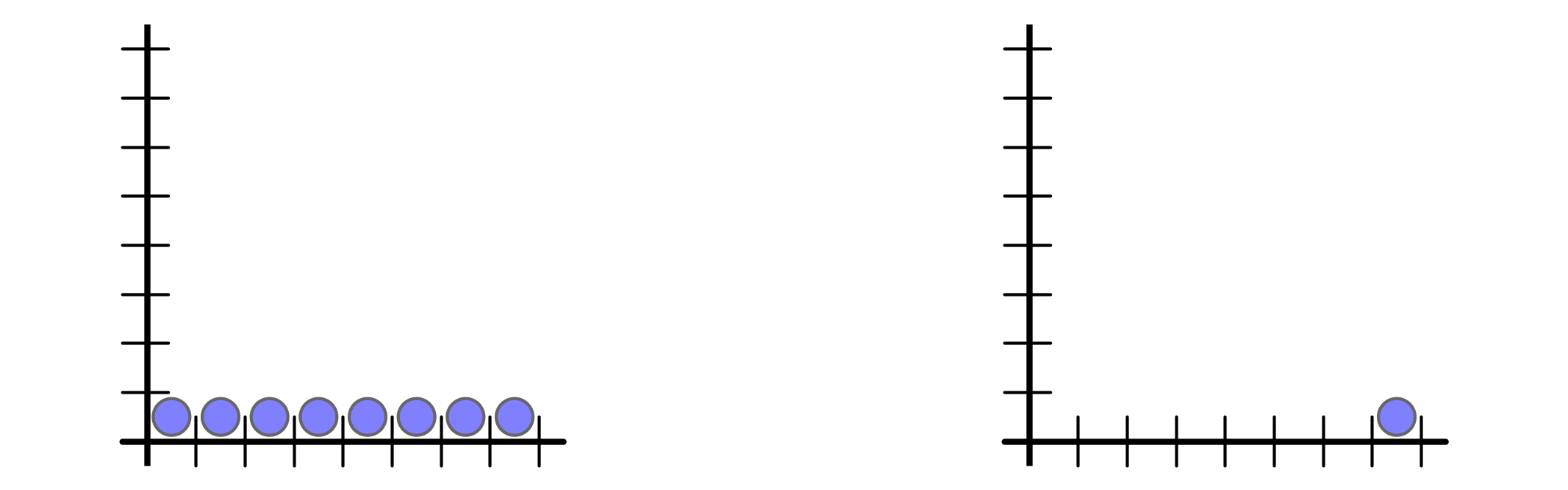But buffer space requirement is $n$ vs $1$

## Global Bursts

AQT parameters do not distinguish ($\rho = 1, \sigma = n - 1$):

• Ex 1 every $n$ rounds, inject $n$ packets into buffer $1$

• Ex 2 every $n$ rounds, injection $1$ packet into buffers $1, 2, \ldots, n$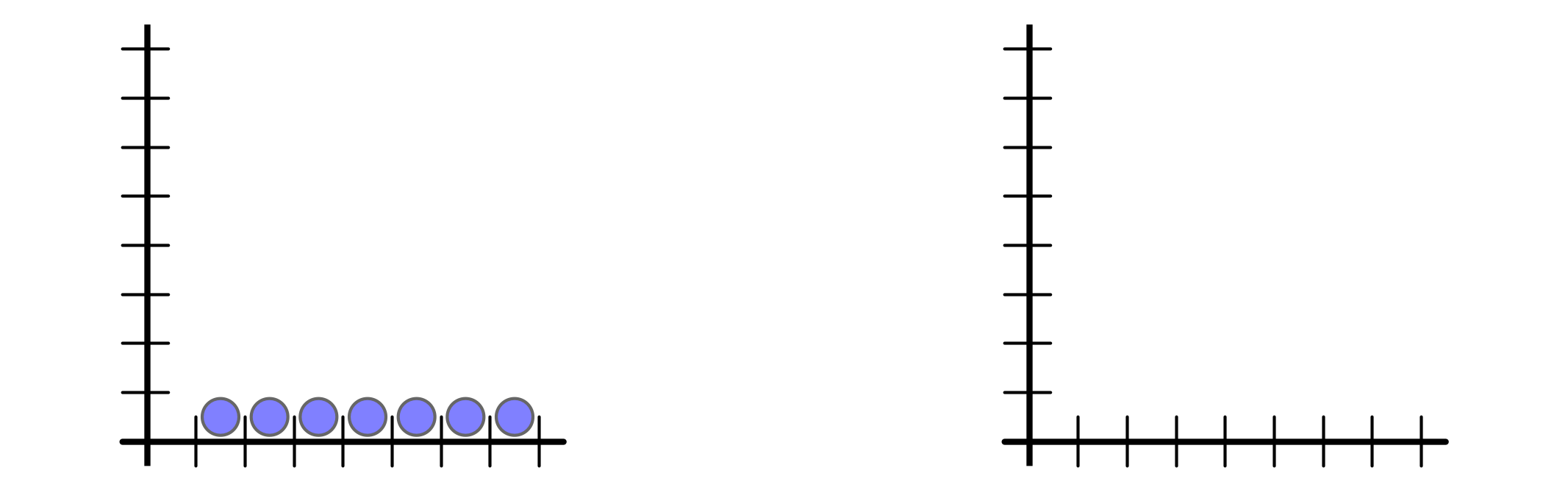But buffer space requirement is $n$ vs $1$

## Global Bursts

AQT parameters do not distinguish ($\rho = 1, \sigma = n - 1$):

• Ex 1 every $n$ rounds, inject $n$ packets into buffer $1$

• Ex 2 every $n$ rounds, injection $1$ packet into buffers $1, 2, \ldots, n$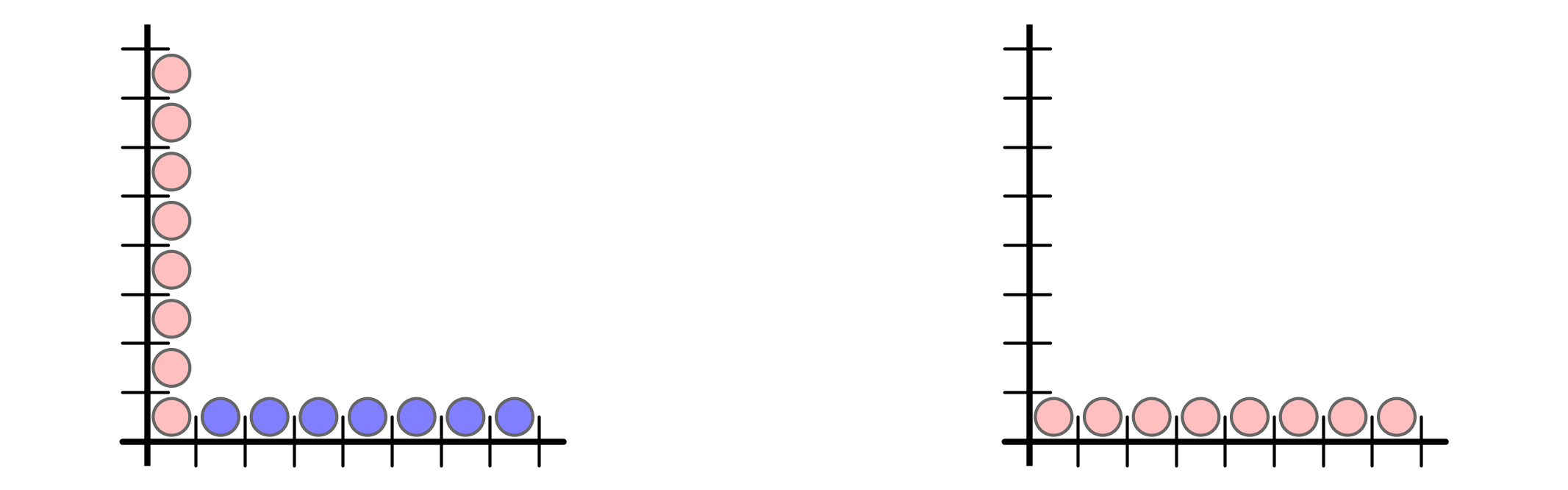But buffer space requirement is $n$ vs $1$

## Proposed Model

• distinguish packets by origin
• local burst parameter $B$
• for every set $S$ of buffers, $t$ consecutive rounds
• number of packets injected into $S$ is at most $B \vert S \vert + \rho t$

Previous examples

• Ex 1 has local burst parameter $B = n - 1$
• Ex 2 has local burst parameter $B = 1$

LBA is strict refinement of AQT model

# Packet Bundling and LBAs

## Issue with Previous Protocol Analyses

General capacities, $C$

• simple protocols defined for $C = 1$
• reasoning about $C > 1$ is subtle
• how many packets should each buffer forward?
• how to deal with “fractional part” of load?
• previous OED analysis was incomplete!

## Packet Bundling Strategy, $C > 1$

• bundle groups of $C$ packets together
• treat bundles as individual packets
• ignore fractional part
• apply protocol for $C = 1$ to bundles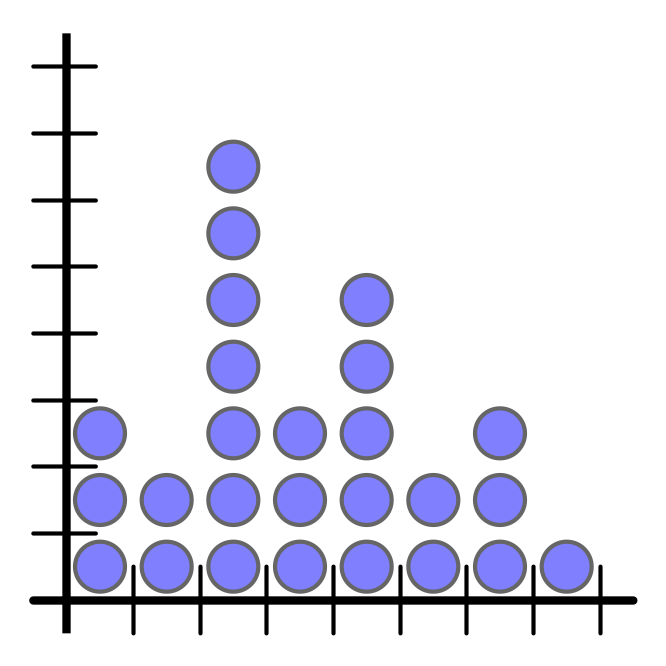## Packet Bundling Strategy, $C > 1$

• bundle groups of $C$ packets together
• treat bundles as individual packets
• ignore fractional part
• apply protocol for $C = 1$ to bundles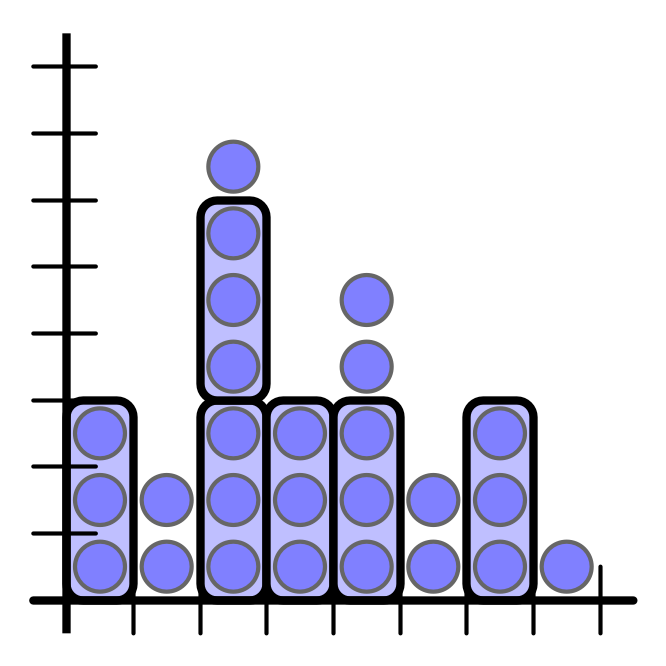## Packet Bundling Strategy, $C > 1$

• bundle groups of $C$ packets together
• treat bundles as individual packets
• ignore fractional part
• apply protocol for $C = 1$ to bundles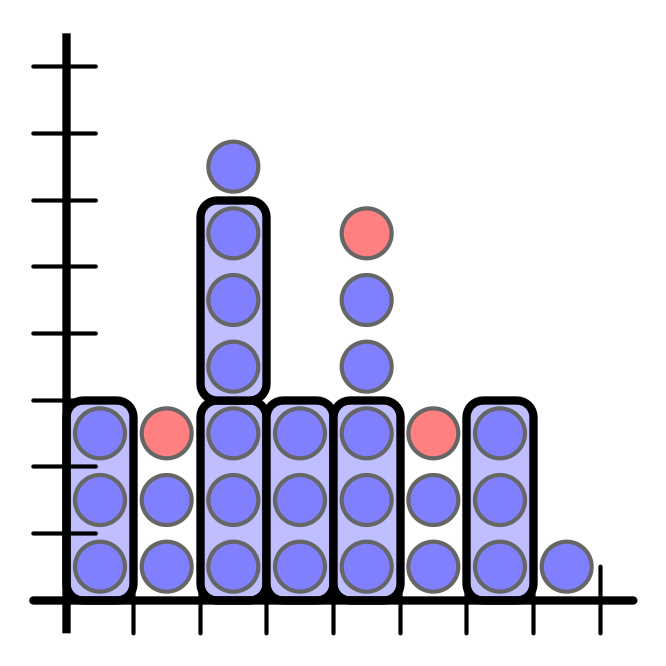## Packet Bundling Strategy, $C > 1$

• bundle groups of $C$ packets together
• treat bundles as individual packets
• ignore fractional part
• apply protocol for $C = 1$ to bundles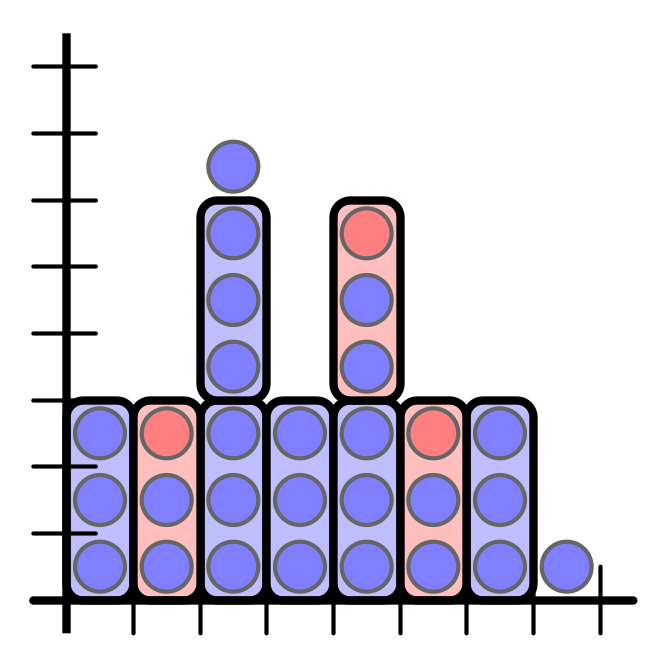## Bundling with an LBA

Theorem 1.

• consider bundling strategy
• original injection has parameters $\rho, \sigma, B$
• then arrival pattern of bundles is an LBA with parameters $\rho/C, \sigma/C, 1 + B / C$

Consequence. Any protocol/analysis for $C = 1$ can be applied to general $C$ as well.

• techniques can also be applied to heterogenous packet sizes

Note. Analogous results for AQT model do not hold.

# Buffer Space Bounds for LBAs

## Local Forwarding vs LBAs

Odd-Even Downhill Forwarding (OED). (Dobrev et al. + Patt-Shamir & R–). Each round, buffer $i$ forwards iff

• $L(i) > L(i+1)$, or
• $L(i) = L(i+1)$ and $L(i)$ is odd

Theorem 2. For any LBA with parameters $\rho \leq 1$, $\sigma, B \geq 1$, the buffer space requirement of OED is $O(B \log n + \sigma)$.

• AQT analysis had $O(\log n + \sigma)$ for $\rho \leq 1$

• Theorem 2 follows from more refined analysis of OED algorithm

## Lower Bounds

Theorem 3. $P$ any (possibly centralized and/or randomized) forwarding protocol, $B, \sigma \geq 1$. Then there is an LBA such that $P$ requires $\Omega(B \log n + \sigma)$ buffer space.

Consequences.

1. OED forwarding is asymptotically optimal for LBAs
2. Locality/buffer space tradeoff disappears in LBA model
• For AQT model (P-S & R– 2019):

For protocols with locality $d$, buffer space $\Theta(1 + \frac 1 d \log n)$ is necessary and sufficient

• For LBA model: local is optimal!

## Next Steps

1. Consider more general topologies
• path with $k$ destinations requires $\Theta(k)$ buffer space (offline/centralized) even without bursts
• local solution for multiple destinations?
2. Semi-synchronous model?
• results for continuous flows (in paper) may be relevant
3. Locality vs Quality for other online problems?